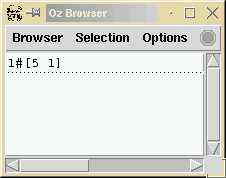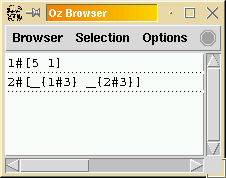## 6.6 Search Predicates

A typical way to solve a CSP in Oz, is to express this CSP as a predicate and invoke the Explorer [Sch97a] [Sch99] with this predicate as an argument.

`{ExploreAll SimpleProblem}`

We explain now how to write such a predicate. It always follows the same pattern.

`declare proc {SimpleProblem Solution}   %% declare variables   X Yin    %% representation of solution   Solution = [X Y]    %% pose constraints of CSP   X::1#9   Y::1#9   X+2*Y =: 7    %% distribution strategy   {FD.distribute ff [X Y]}end`

`SimpleProblem` is a predicate that defines what is a `Solution`: a solution is a list `[X Y]` of two integers satisfying the equation `X+2*Y =: 7`. It invokes the predefined distribution strategy `{FD.distribute ff [X Y]}`, where `ff` means first-fail i.e. ``choose the variable with the fewer remaining possible values, and then try each one of these values in increasing order''.

`{ExploreAll SimpleProblem}` produces the following search tree in the Explorer:clicking on the first green diamond (a solution) shows the following in the browser:We can also click on the second blue circle (a choice point) to observe the partial information at that point:For a thorough tutorial on constraint programming in Oz, we recommend again to read [SS99].

Denys Duchier
Version 1.2.0 (20010221)